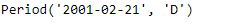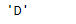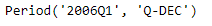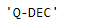# Python | Pandas Period.freqstr

• Last Updated : 06 Jan, 2019

Python is a great language for doing data analysis, primarily because of the fantastic ecosystem of data-centric python packages. Pandas is one of those packages and makes importing and analyzing data much easier.

Pandas` Period.freqstr` attribute returns the string alias of the Time series frequency applied on the given Period object.

Attention geek! Strengthen your foundations with the Python Programming Foundation Course and learn the basics.

To begin with, your interview preparations Enhance your Data Structures concepts with the Python DS Course. And to begin with your Machine Learning Journey, join the Machine Learning - Basic Level Course

Syntax : Period.freqstr

Parameters : None

Return : string

Example #1: Use `Period.freqstr` attribute to find the string alias of the time series frequency applied on the given Period object.

 `# importing pandas as pd``import` `pandas as pd`` ` `# Create the Period object``prd ``=` `pd.Period(freq ``=``'D'``, year ``=` `2001``, month ``=` `2``, day ``=` `21``)`` ` `# Print the Period object``print``(prd)`

Output :Now we will use the `Period.freqstr` attribute to find the string alias of the frequency applied on prd object.

 `# return the string alias of the frequency``prd.freqstr`

Output :As we can see in the output, the `Period.freqstr `attribute has returned ‘D’ indicating that the time series frequency applied on the given object was day.

Example #2: Use `Period.freqstr` attribute to find the string alias of the time series frequency applied on the given Period object.

 `# importing pandas as pd``import` `pandas as pd`` ` `# Create the Period object``prd ``=` `pd.Period(freq ``=``'Q'``, year ``=` `2006``, quarter ``=` `1``)`` ` `# Print the object``print``(prd)`

Output :Now we will use the `Period.freqstr` attribute to find the string alias of the frequency applied on prd object.

 `# return the string alias of the frequency``prd.freqstr`

Output :As we can see in the output, the `Period.freqstr `attribute has returned ‘Q-DEC’ indicating that the time series frequency applied on the given object was the end quarter of the year.

My Personal Notes arrow_drop_up